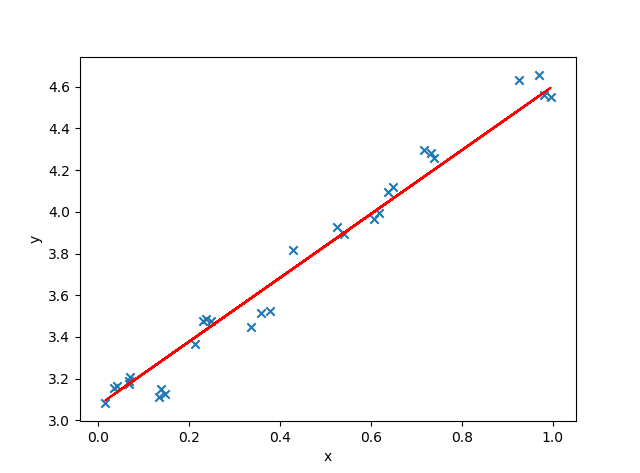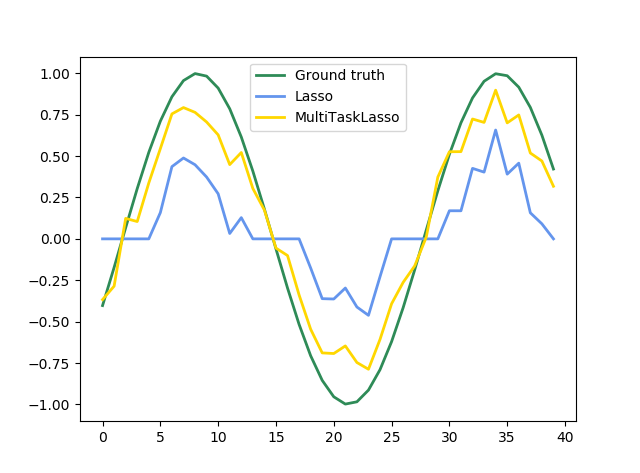# python机器学习库sklearn——Lasso回归（L1正则化）

python数据挖掘系列教程

## Lasso

The Lasso 是估计稀疏系数的线性模型。 它在一些情况下是有用的，因为它倾向于使用具有较少参数值的情况，有效地减少给定解决方案所依赖变量的数量。 因此，Lasso 及其变体是压缩感知领域的基础。 在一定条件下，它可以恢复一组非零权重的精确集。

$\underset{w}{min\phantom{\rule{thinmathspace}{0ex}}}\frac{1}{2{n}_{samples}}||Xw-y|{|}_{2}^{2}+\alpha ||w|{|}_{1}$

lasso 估计解决了加上罚项$\alpha ||w|{|}_{1}$$\alpha ||w||_1$的最小二乘法的最小化，其中，$\alpha$$\alpha$ 是一个常数，$||w|{|}_{1}$$||w||_1$是参数向量的 ${\ell }_{1}-norm$$\ell_1-norm$ 范数。

Lasso 类的实现使用了 coordinate descent （坐标下降算法）来拟合系数。

## 设置正则化参数

scikit-learn 通过交叉验证来公开设置 Lasso中$\alpha$$\alpha$ 参数的对象: LassoCV 和 LassoLarsCV。 LassoLarsCV 是基于下面解释的 最小角回归 算法。

$\alpha$$\alpha$ 和 SVM 的正则化参数$C$$C$之间的等式关系是 $\alpha =1/C$$\alpha = 1 / C$ 或者 $\alpha =1/\left({n}_{samples}\ast C\right)$$\alpha = 1 / (n_{samples} * C)$，并依赖于估计器和模型优化的确切的目标函数。

## 主参数设置

alpha : float, 可选，默认 1.0。当 alpha 为 0 时算法等同于普通最小二乘法，可通过 Linear Regression 实现，因此不建议将 alpha 设为 0.

fit_intercept : boolean

normalize : boolean, 可选, 默认 False

copy_X : boolean, 可选, 默认 True

precompute : True | False | array-like, 默认=False

max_iter : int, 可选

tol : float, 可选

warm_start : bool, 可选

positive : bool, 可选

selection : str, 默认 ‘cyclic’

random_state : int, RandomState instance, 或者 None (默认值)
pseudo random number generator 用来产生随机 feature 进行更新时需要用的

seed。仅当 selection 为 random 时才可用。

## 代码实现

import numpy as np # 快速操作结构数组的工具
import matplotlib.pyplot as plt  # 可视化绘制
from sklearn.linear_model import Lasso,LassoCV,LassoLarsCV   # Lasso回归,LassoCV交叉验证实现alpha的选取，LassoLarsCV基于最小角回归交叉验证实现alpha的选取

# 样本数据集，第一列为x，第二列为y，在x和y之间建立回归模型
data=[
[0.067732,3.176513],[0.427810,3.816464],[0.995731,4.550095],[0.738336,4.256571],[0.981083,4.560815],
[0.526171,3.929515],[0.378887,3.526170],[0.033859,3.156393],[0.132791,3.110301],[0.138306,3.149813],
[0.247809,3.476346],[0.648270,4.119688],[0.731209,4.282233],[0.236833,3.486582],[0.969788,4.655492],
[0.607492,3.965162],[0.358622,3.514900],[0.147846,3.125947],[0.637820,4.094115],[0.230372,3.476039],
[0.070237,3.210610],[0.067154,3.190612],[0.925577,4.631504],[0.717733,4.295890],[0.015371,3.085028],
[0.335070,3.448080],[0.040486,3.167440],[0.212575,3.364266],[0.617218,3.993482],[0.541196,3.891471]
]

#生成X和y矩阵
dataMat = np.array(data)
X = dataMat[:,0:1]   # 变量x
y = dataMat[:,1]   #变量y

# ========Lasso回归========
model = Lasso(alpha=0.01)  # 调节alpha可以实现对拟合的程度
# model = LassoCV()  # LassoCV自动调节alpha可以实现选择最佳的alpha。
# model = LassoLarsCV()  # LassoLarsCV自动调节alpha可以实现选择最佳的alpha
model.fit(X, y)   # 线性回归建模
print('系数矩阵:\n',model.coef_)
print('线性回归模型:\n',model)
# print('最佳的alpha：',model.alpha_)  # 只有在使用LassoCV、LassoLarsCV时才有效
# 使用模型预测
predicted = model.predict(X)

# 绘制散点图 参数：x横轴 y纵轴
plt.scatter(X, y, marker='x')
plt.plot(X, predicted,c='r')

# 绘制x轴和y轴坐标
plt.xlabel("x")
plt.ylabel("y")

# 显示图形
plt.show()## 多任务回归

MultiTaskLasso 是一个估计多元回归稀疏系数的线性模型： $y$$y$是一个 $\left({n}_{samples},{n}_{tasks}\right)$$(n_{samples}, n_{tasks})$ 的二维数组，其约束条件和其他回归问题（也称为任务）是一样的，都是所选的特征值。

$\underset{w}{min\phantom{\rule{thinmathspace}{0ex}}}\frac{1}{2{n}_{samples}}||XW-Y|{|}_{Fro}^{2}+\alpha ||W|{|}_{21}$

$||A|{|}_{Fro}=\sqrt{\sum _{ij}{a}_{ij}^{2}}$

$||A|{|}_{21}=\sum _{i}\sqrt{\sum _{j}{a}_{ij}^{2}}$

MultiTaskLasso 类的实现使用了坐标下降作为拟合系数的算法。

import matplotlib.pyplot as plt
import numpy as np
from sklearn.linear_model import MultiTaskLasso, Lasso

rng = np.random.RandomState(42)
# ===========================产生模拟样本数据=========================
# 用随机的频率、相位产生正弦波的二维系数
n_samples, n_features, n_tasks = 100, 30, 40  # n_samples样本个数，n_features特征个数，n_tasks估计值的个数
n_relevant_features = 5 # 自定义实际有用特征的个数
coef = np.zeros((n_tasks, n_features)) # 系数矩阵的维度

times = np.linspace(0, 2 * np.pi, n_tasks)
for k in range(n_relevant_features):
coef[:, k] = np.sin((1. + rng.randn(1)) * times + 3 * rng.randn(1)) # 自定义数据矩阵，用来生成模拟输出值

X = rng.randn(n_samples, n_features)  # 产生随机输入矩阵
Y = np.dot(X, coef.T) + rng.randn(n_samples, n_tasks) # 输入*系数+噪声=模拟输出
# ==============================使用样本数据训练系数矩阵============================
coef_lasso_ = np.array([Lasso(alpha=0.5).fit(X, y).coef_ for y in Y.T])
coef_multi_task_lasso_ = MultiTaskLasso(alpha=1.).fit(X, Y).coef_  # 多任务训练

# #############################################################################
# Plot support and time series
fig = plt.figure(figsize=(8, 5))
plt.subplot(1, 2, 1)
plt.spy(coef_lasso_)
plt.xlabel('Feature')
plt.ylabel('Time (or Task)')
plt.text(10, 5, 'Lasso')
plt.subplot(1, 2, 2)
plt.spy(coef_multi_task_lasso_)
plt.xlabel('Feature')
plt.ylabel('Time (or Task)')
plt.text(10, 5, 'MultiTaskLasso')
fig.suptitle('Coefficient non-zero location')

feature_to_plot = 0
plt.figure()
lw = 2
plt.plot(coef[:, feature_to_plot], color='seagreen', linewidth=lw,
label='Ground truth')
plt.plot(coef_lasso_[:, feature_to_plot], color='cornflowerblue', linewidth=lw,
label='Lasso')
plt.plot(coef_multi_task_lasso_[:, feature_to_plot], color='gold', linewidth=lw,
label='MultiTaskLasso')
plt.legend(loc='upper center')
plt.axis('tight')
plt.ylim([-1.1, 1.1])
plt.show()